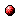# Model predictive control for stochastic max-plus linear systems with chance constraints

Reference:
J. Xu, T. van den Boom, and B. De Schutter, "Model predictive control for stochastic max-plus linear systems with chance constraints," IEEE Transactions on Automatic Control, vol. 64, no. 1, pp. 337-342, 2019.

Abstract:
The topic of this paper is model predictive control (MPC) for max-plus linear systems with stochastic uncertainties the distribution of which is supposed to be known. We consider linear constraints on the inputs and the outputs. Due to the uncertainties, these linear constraints are formulated as probabilistic or chance constraints, i.e., the constraints are required to be satisfied with a predefined probability level. The proposed chance constraints can be equivalently rewritten into a max-affine (i.e., the maximum of affine terms) form if the linear constraints are monotonically nondecreasing as a function of the outputs. Based on the resulting max-affine form, two methods are developed for solving the chance-constrained MPC problem for stochastic max-plus linear systems. Method 1 uses Boole's inequality to convert the multivariate chance constraint into univariate chance constraints for which the probability can be computed more efficiently. Method 2 employs Chebyshev's inequality and transforms the chance constraint into linear constraints on the inputs. The simulation results for a production system example show that the two proposed methods are faster than the Monte Carlo simulation method and yield lower closed-loop costs than the nominal MPC method.

Downloads:Online version of the paperCorresponding technical report: pdf file (145 KB)
Note: More information on the pdf file format mentioned above can be found here.

Bibtex entry:

@article{Xuvan:17-016,
author={J. Xu and T. van den Boom and B. {D}e Schutter},
title={Model predictive control for stochastic max-plus linear systems with chance constraints},
journal={IEEE Transactions on Automatic Control},
volume={64},
number={1},
pages={337--342},
year={2019},
doi={10.1109/TAC.2018.2849570}
}

Go to the publications overview page.

This page is maintained by Bart De Schutter. Last update: August 12, 2020.